Courses

# Solutions of Reflection of Light (Page No- 199) - Physics By Lakhmir Singh, Class 10 Class 10 Notes | EduRev

## Class 10 : Solutions of Reflection of Light (Page No- 199) - Physics By Lakhmir Singh, Class 10 Class 10 Notes | EduRev

The document Solutions of Reflection of Light (Page No- 199) - Physics By Lakhmir Singh, Class 10 Class 10 Notes | EduRev is a part of the Class 10 Course Class 10 Physics Solutions By Lakhmir Singh & Manjit Kaur.
All you need of Class 10 at this link: Class 10

Question 18: An object 3 cm high is placed at a distance of 10 cm in front of a converging mirror of focal length 20 cm. Find the position, nature and size of the image formed.

Solution : Given : h= 3cm, u = -10cm, f = -20cm,

We know that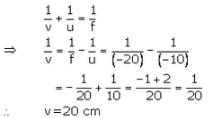The image is formed at a distance of 20 cm behind the mirror.

And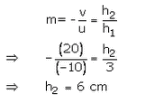Image is 6 cm in size , virtual and erect.

Question 19: A concave mirror has a focal length of 4 cm and an object 2 cm tall is placed 9 cm away from it. Find the nature, position and size of the image formed.

Solution : h1 = 2cm, u =-9cm, f = -4cm

We know that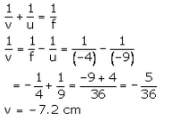The image is formed at a distance 7.2 cm in front of the mirror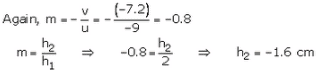So, image is 1.6 cm in size, real and inverted.

Question 20: When an object is placed 20 cm from a concave mirror, a real image magnified three times is formed. Find :
(a) the focal length of the mirror.
(b) Where must the object be placed to give a virtual image three times the height of the object ?

Solution : Given : u = -20cm.m = - 3, for the real image

(a) We know that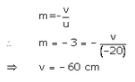We have,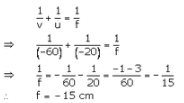(b) For virtual image m = 3, and f = -15crn

We know that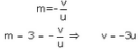We have,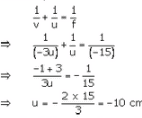So object should be placed 10cm from the concave mirror.

Question 21: A dentist’s mirror has a radius of curvature of 3 cm. How far must it be placed from a small dental cavity to give a virtual image of the cavity that is magnified five times ?

Solution : R = -3crn (concave mirror)
m = 5 (virtual image)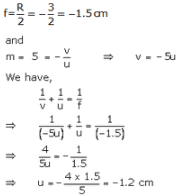The mirror should be placed 1.2cm away from the dental cavity.

Question 22: A large concave mirror has a radius of curvature of 1.5 m. A person stands 10 m in front of the mirror. Where is the person’s image ?

Solution : R = -1.5crn (concave mirror)
u = -10 cm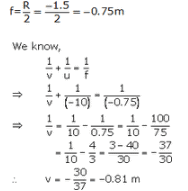The perscn's image will be 0.81 m in front of ccncave mirror.

Question 23: An object of 5.0 cm size is placed at a distance of 20.0 cm from a converging mirror of focal length 15.0 cm. At what distance from the mirror should a screen be placed to get the sharp image ? Also calculate the size of the image.

Solution : h1= 5.0cm. u = -20cm. f = -15cm. v=?. h2=?

We know that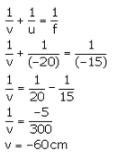The screen should be placed 60cm in front of the mirror.

And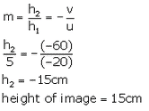Question 24: A concave mirror produces three times enlarged virtual image of an object placed at 10 cm in front of it. Calculate the radius of curvature of the mirror.

Solution : m = 3 (virtual image)
u =-10cm
R = ?

We know that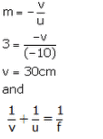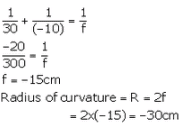Question 25: A bright object 50 mm high stands on the axis of a concave mirror of focal length 100 mm and at a distance of 300 mm from the concave mirror. How big will the image be ?

Solution : h1 = 50mm. f = -100mm. u = -300mm. h1=?

We have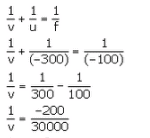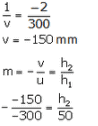The Image will be 25 mm high.

Question 26: How far should an object be placed from the pole of a converging mirror of focal length 20 cm to form a real image of the size exactly 1/4th the size of the object ?

Solution : f = -20cm, m = -1/4 (real image)

We know that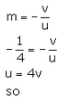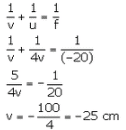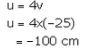The object should be placed 100 cm to the left of the mirror.

Question 27: When an object is placed at a distance of 50 cm from a concave spherical mirror, the magnification produced is, -1/2 Where should the object be placed to get a magnification of, -1/5?

Solution :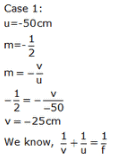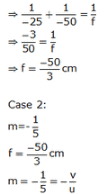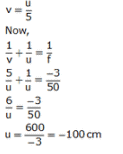Question 28: An object is placed (a) 20 cm, (b) 4 cm, in front of a concave mirror of focal length 12 cm. Find the nature and position of the image formed in each case.

Solution :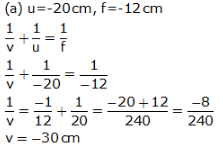The image is formed at a distance of 30 cm in front of the mirror. The image is real and inverted.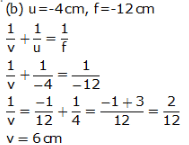The image is formed at a distance of 6 cm behind the mirror. The image is virtual and erect.

Question 29: A concave mirror produces a real image 1 cm tall of an object 2.5 mm tall placed 5 cm from the mirror. Find the position of the image and the focal length of the mirror.

Solution : h2 = 1cm = 10mm (real image), h1 = 2.5mm. u = -5cm = -50mm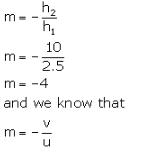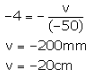The image is formed 20 cm in front of the mirror.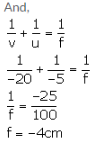Question 30: A man holds a spherical shaving mirror of radius of curvature 60 cm, and focal length 30 cm, at a distance of 15 cm, from his nose. Find the position of image, and calculate the magnification.

Solution :

Radius of curvature, R = -60cm (concave mirror)
f = -30cm. u = -15cm

We have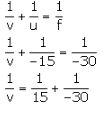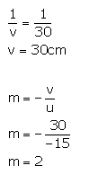So. the the image is formed 30 cm behind the mirror and the magnification is +2.

Question 31: (a) An object is placed just outside the principal focus of concave mirror. Draw a ray diagram to show how the image is formed, and describe its size, position and nature.
(b) If the object is moved further away from the mirror, what changes are there in the position and size of the image ?
(c) An object is 24 cm away from a concave mirror and its image is 16 cm from the mirror. Find the focal length and radius of curvature of the mirror, and the magnification of the image.

Solution :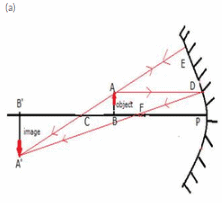The image is real inverted and magnified It is formed beyond the centre of curvature of the mirror.
(b) If the object is moved further away from the mirror. the image is tamed nearer to the mirror and its size goes on decreasing.
(c) u = -24cm
v = -1.6cm
f = ?.R = ?.m = ?
weknow that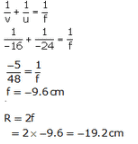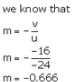Offer running on EduRev: Apply code STAYHOME200 to get INR 200 off on our premium plan EduRev Infinity!

94 docs

,

,

,

,

,

,

,

,

,

,

,

,

,

,

,

,

,

,

,

,

,

,

,

,

;